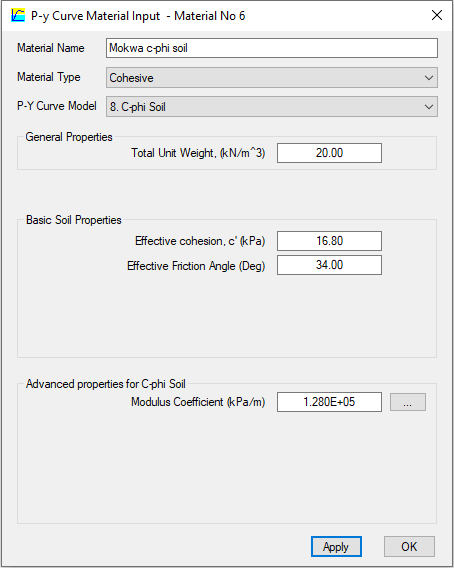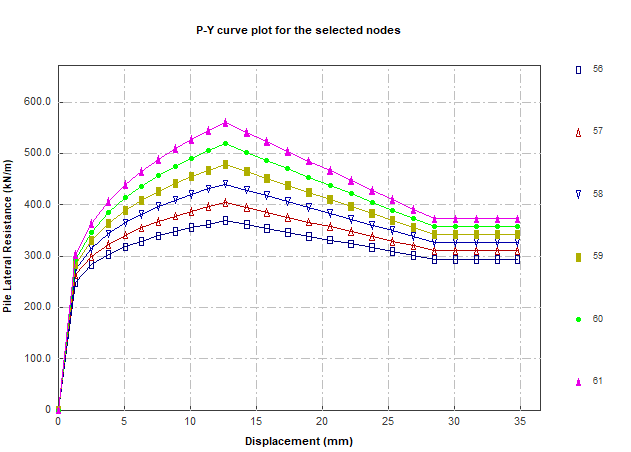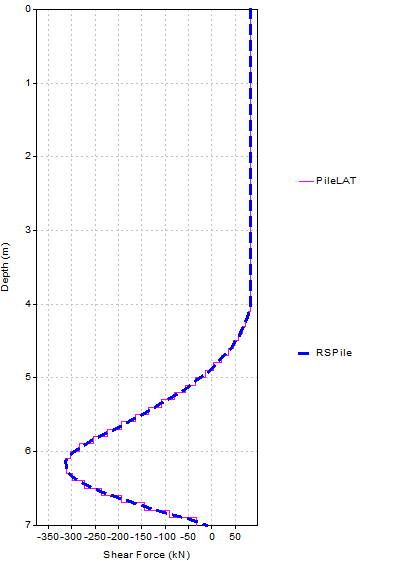top of page

# A case study for laterally loaded piles in c-phi soils using the PileLAT program

### 1.0 Introduction

This blog reports a case study for laterally loaded piles in soils with both cohesion and friction (c-phi soils) using the PileLAT program. The example problem from Mokwa et al. (2000) with the title of "Development of p-y curves for partly saturated silts and clays" is used in this case study. The configuration of the drilled shaft subject to a lateral force at the top is extracted from the paper and shown as below.Drilled shaft under horizontal load the pile head (after Mokwa et al. 2000)

### 2.0 Theoretical background of p-y curve model for c-phi soil

The p-y curve model for c-phi soil model based on the recommendation from Evans and Duncan (1982) is adopted in the PileLAT program. The typical p-y curve is shown in the figure below.The p-y curve for c-phi soils consists of the following four components:

• Initial linear section between the original point and point k with slope of Ks=kpy x Z where kpy is the modulus coefficient and Z is the point depth.

• Parabolic section between points k and m defined by the relationship of• Linear section between points m and u with slope of m• Last linear section with the flat line beyond point uThe following equations are used to calculate the control points along the p-y curve:Pst is the ultimate resistance of sand at the shallow depths and is determined according to the following relationship:Psd is the ultimate resistance of sand at deep depths and is determined according to the following equation:wherePc is the cohesion component, which will be the smaller of the values given by the equations below:where### 3.0 Analysis results with using the PileLAT programThe p-y curve input dialog for the example mentioned in Section 1 is shown in the left figure. Note that the modulus coefficient used to calculate the initial stiffness of p-y curve is adopted to be 128 MN/m^3 for this case as it is not clear which value was adopted in the paper by Mokwa et al. (2000). For the same reason, the elastic modulus of the shaft material is assumed to be 25 GPa in this case study.

The applied horizontal at 4 m above the ground surface is 82 kN and the model geometry in PileLAT is shown as below.The analysis results of deflection, bending moment and shear force from the PileLAT program are shown as below.The typical p-y curves for c-phi soils at various depths below the ground surface are shown in the figure below.The detailed p-y curve calculation information can be reported by the built-in PYAnyTool function and the screen shot is shown as below:### 4.0 Comparison results with the RSPile program

RSPile is also used to analyze this case to compare the results from the PileLAT program. The following figures (deflection and bending moment) provides the result comparisons between those two different programs.The results of the shear force distribution along the pile length from both the PileLAT and RSPile programs are shown in the figure below. It can be seen that the results from the PileLAT program (Pink color) are almost the same as the results from the RSPile program (Blue color).### 5.0 Conclusions

A case study has been carried out using the PileLAT program for the example problem reported in Mokwa et al. (2000). The c-phi p-y curve model is adopted for foundation soil and a cantilevered pile subject to a horizontal shear force at the pile head is analyzed. The analysis results, including deflection, bending moment, and shear force, obtained from the PileLAT program were compared to those from the RSPile program, and it was found that the differences between the two programs were negligible.

602 views